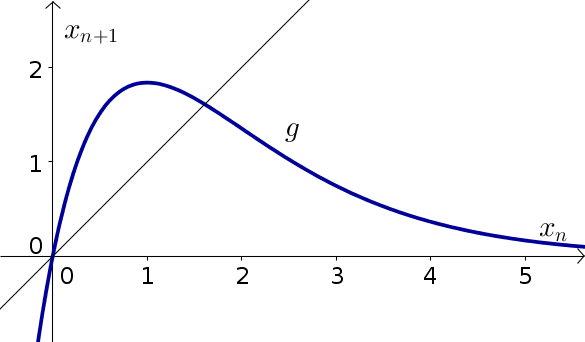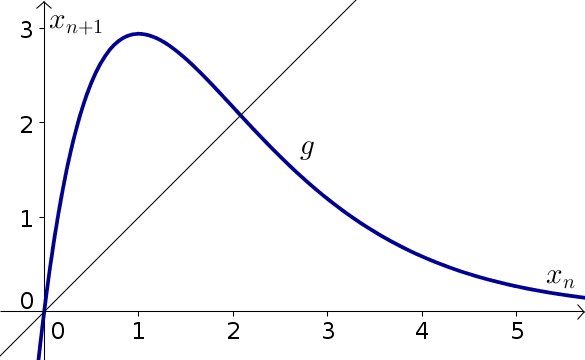# Math Insight

### Elementary discrete dynamical systems biology problems, part 2

#### Problem 1

Let $x_n$ be the number of fish in generation $n$ in a lake. We'll model the evolution of the fish using Ricker's model of the form \begin{align*} x_{n+1} = 5x_n e^{-x_n}, \end{align*}

The equilibria of this model are $x_n=0$ and $x_n= \ln(5)$.

1. Calculate the stability of the equilibria using calculus.
2. Confirm the stability through cobwebbing the below graph.
3. For any positive initial fish population $x_0$, what will happen to the fish population $x_n$ as $n$ gets large?#### Problem 2

Let $x_n$ be the number of fish in generation $n$ in a lake. We'll model the evolution of the fish using Ricker's model of the form \begin{align*} x_{n+1} = 8x_n e^{-x_n}, \end{align*}

The equilibria of this model are $x_n=0$ and $x_n= \ln(8)$.

1. Calculate the stability of the equilibria using calculus.
2. Confirm the stability through cobwebbing the below graph.
3. If the initial fish population $x_0$ is close to (but not equal to) $\ln(8)$, what will happen to the fish population $x_n$ as $n$ increases?#### Problem 3

A population of squirrels would be increasing at rate of 50% per year, except that due to a scarcity of acorns, there is only enough food to support 300 squirrels. Therefore, if $s_t$ is the number of squirrels in year $t$, the population evolves according to the dynamical system \begin{align*} s_{t+1} - s_{t} = 0.5 s_t\left(1 - \frac{s_t}{300}\right). \end{align*}

Calculate the equilibria of the dynamical system and their stability. If the initial population is $s_0=400$, what will happen to the population after a long time?

#### Problem 4

A population of squirrels would be increasing at rate of $r$ per year, except that due to a scarcity of acorns, there is only enough food to support $N$ squirrels. Therefore, if $s_t$ is the number of squirrels in year $t$, the population evolves according to the dynamical system \begin{align*} s_{t+1} - s_{t} = r s_t\left(1 - \frac{s_t}{N}\right). \end{align*}

1. Calculate the equilibria of the dynamical system.
2. If $r=1.5$, determine the stability of the equilibria.
3. If $r=1.5$, $N=500$, and the initial population is $s_0=400$, what will happen to the population after a long time?
4. If $r=3$, determine the stability of the equilibria.
5. For what range of $r$ is there a stable nonzero equilibrium?

#### Problem 5

A population of deer is growing exponentially so that in each year the population increases by 20%. In order to keep the population from exploding, a number of deer is harvested annually that is proportional to the square of the population. If $d_t$ is the number of deer in year $t$, the population follows the dynamical system \begin{align*} d_{t+1}=1.2 d_t - c d_t^2 \end{align*} where $c$ is a positive parameter that determines the amount of harvesting.

1. Find the equilibria of this system.
2. Calculate the stability of the equilibria.
3. How does the stability of the equilibria depend on the parameter $c$?
4. For what value of $c$ is there a stable equilibrium of 200 deer?

#### Problem 6

A population of locusts is growing out of control, so that the number of locusts is quadupling each year. In attempt to limit the population, each year a number of locusts is killed that is proportional to the square of the population. If $l_t$ is the number of locusts in year $t$, the population follows the dynamical system \begin{align*} l_{t+1}=4 l_t - c l_t^2 \end{align*} where $c$ is a positive parameter that determines the amount of harvesting.

1. Find the equilibria of this system.
2. Calculate the stability of the equilibria.
3. How does the stability of the equilibria depend on the parameter $c$?
4. Will this strategy succeed in bringing the number of locusts to a stable small population?

One you have worked on a few problems, you can compare your solutions to the ones we came up with.